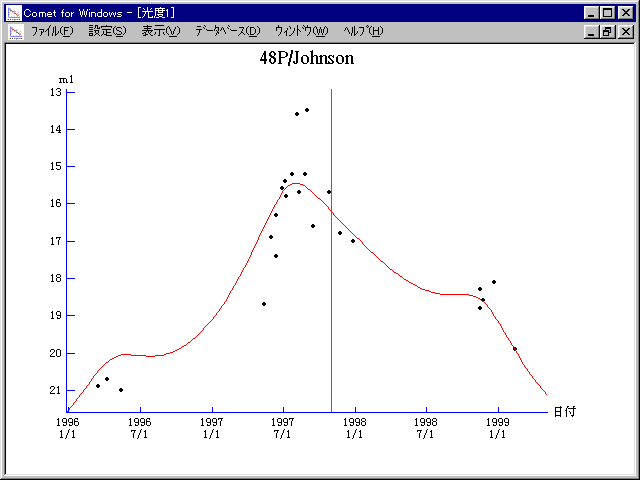# \$B%8%g%s%=%sWB@1(B

48P/Johnson (1997)###\$B50F;MWAG(B

```   The following improved orbital elements by Kenji Muraoka,
are from 234 observation1 1949 to 1999, including 9 Planets,
Moon and 5 minor planets perturbations and non-gravitational
effect of style II.  The mean residual is +/- 0.81 arc seconds.

Epoch  =  1997 Nov.  8.0  TT       JDT = 2450760.5
T  =  1997 Oct. 31.84877       +/- 0.00072 (m.e.) TT
Peri. =  207.99089                +/- 0.00025
Node  =  117.35386                +/- 0.00005   (2000.0)
Incl. =   13.66435                +/- 0.00002
q  =    2.3083073              +/- 0.0000010 AU
e  =    0.3672592              +/- 0.0000003
a  =    3.6481085              +/- 0.0000006 AU
n  =    0.14144984             +/- 0.00000004
P  =    6.968                  +/- 0.0000018  years
A1  =   +0.711                  +/- 0.021
A2  =   -0.02396                +/- 0.00069
```

###\$B@1?^(B1997\$BG/(B 2\$B7n(B 1\$BF|!A(B1998\$BG/(B12\$B7n(B13\$BF|(B

###\$B8wEYJQ2=(B

```        m1 = 9.0 + 5 log\$B&\$(B + 15.0 log r
```##### \$B50F;MWAG\$OB<2,7r<#;a\$N7W;;\$K\$h\$k\$b\$N\$G\$9!#(B \$B@1?^\$O(B StellaNavigator Ver.2.0 for Windows (\$B%"%9%H%m%"!<%D(B \$BJTCx(B / \$B%"%9%-!<=PHG6I4)(B) \$B\$G:n@.\$7\$?\$b\$N\$G\$9!#(B \$B8wEY%0%i%U\$O(BComet for Windows\$B\$G:n@.\$7\$?\$b\$N\$G\$9!#(B